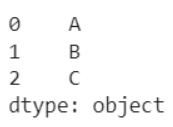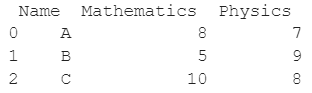# What Is The Pandas Library?

Pandas is a library for data science. It is an open-source, library that provides high-performance, easy-to-use data structures and data analysis tools for the Python programming language.

It is mainly used for working with data sets in a convenient and efficient manner.

The name Pandas originates from the term Panel Data which is a special subset of data in the field of statistics and econometrics.

Since Pandas is an open-source Python library, the source code is openly available at this GitHub repository https://github.com/pandas-dev/pandas.

• It provides a rich set of built-in functions and tools

• Data representation and Manipulation is quite easy

• Compatible to Python

• Efficient for data filtration

## History of Pandas

The Pandas was developed by Wes McKinney in 2008 and later on released as open source. The summarized history of pandas is in the below table.

Year Releases
2008 Development Started
2009 It becomes open source
2012 The first edition released
2015 It becomes NumFOCUS sponsored project
2018 First in-person core developer sprint

## Use cases of Pandas

The Python Pandas library helps a lot in making your data set flexible and customizable.

Pandas is usually employed for the task of data analysis and data pre-processing. Both of these are very crucial stages in the field of data science.

Data analysis involves any sort of operation that helps us to extract information from given data in order to make deductions or future decisions. Some of these operations are loading, cleaning, merging, grouping, aggregating, transforming, alignment, and reshaping data

Pandas is usually preferred over other libraries providing similar functionality because working with Pandas is fast, simple, and more expressive than other tools.

Pandas library is often compared to excel sheets due to its data handling feature. A lot of features in excel sheets are available in the pandas as well.

A striking difference between the two is that excel sheets could easily get very inconvenient to work with as the size of the data set increases. However, this is not the case with Pandas as it is exceptionally versatile when it comes to handling large amounts of data. Code that would otherwise require multiple lines in normal Python could instead be implemented with just a few lines using the Pandas library.

## Installing Pandas in Windows

To install Pandas, you will need to have pip on your system, if it has Windows OS, or pip3, if it has MacOS. To install it, go to your terminal and run the following command:

``pip install pandas``

## Installing Pandas in MacOS

You can use the command in case you have macOS.

``pip3 install pandas``

## Importing Pandas in Python code

To start working with Pandas, we first need to import it. Below is the code snippet that allows us to do so :

``import pandas as pd``

## Data Structures in Pandas

The core data structures of Pandas are Series and DataFrames that allow the user to work with data in an efficient manner. Let us look at them one by one next.

## Series in Pandas

A Pandas series is a one-dimensional array-like structure used for storing and working with data. Series are the most versatile type of data structure in Pandas.

You can use a series to represent a time series, an ordered set, an indexed column, etc. A lot of operations can be performed on the Pandas series. Obviously, one of the first steps to learn before using a Pandas Series is to create it.

Let us understand this operation with the help of an example. Consider the following Pandas Series containing 3 elements as characters A, B, and C.Code snippet for generating the above Pandas Series :

### Python 3 Code :

``````import pandas as pd
# List for our data
data = ['A', 'B', 'C']
# Series for the list
s = pd.Series(data)
# Printing the Series
print(s)``````

## Pandas DataFrames

A Pandas DataFrame is another core data structure of the Python Pandas library. It is a two-dimensional structure usually used to represent tabular data.

It can be stored with labeled axes. Obviously, one of the first steps to learn before using a Pandas DataFrame is to create it.

Let us understand this operation with the help of an example. Consider the following DataFrame containing 3 students with names A, B, and C and their corresponding marks (out of 10) for two subjects, Mathematics and Physics.Code snippet for generating the above DataFrame:

### Python 3 Code :

``````import pandas as pd
# Dictionary for our data
data = {'Name' : ['A', 'B', 'C'], 'Mathematics' : [8, 5, 10], 'Physics' : [7, 9, 8]}
# DataFrame for the dictionary
df = pd.DataFrame(data)
# Printing the DataFrame
print(df)``````

Here, data is a dictionary, we created to initialize the DataFrame. For this, we use the DataFrame() function of the Pandas library which takes the dictionary as an argument and returns the required DataFrame.

## Conclusion

In this topic, we have learned what the Python Pandas library is, its uses, advantages, and the two most important data structures used in Pandas - Series and DataFrames, following a running example of test scores of students in different subjects, thus giving us an intuition of how this concept could be applied in real-world situations.

Trending